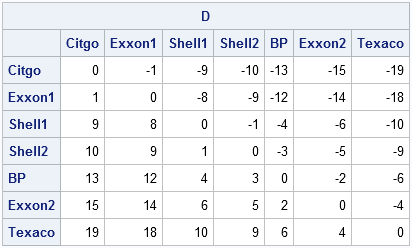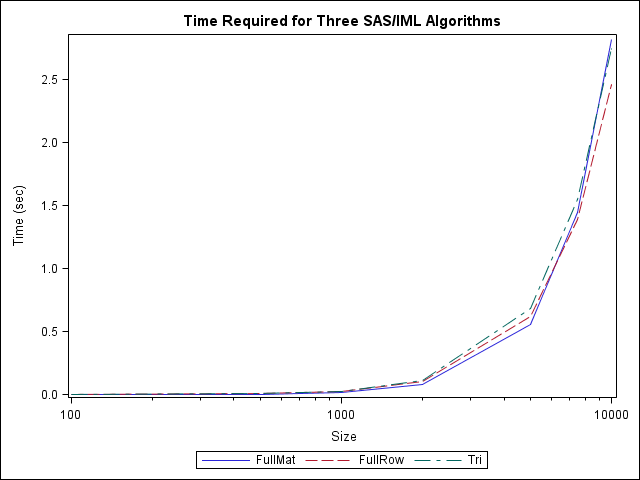The other day, someone asked me how to compute a matrix of pairwise differences for a vector of values. The person asking the question was using SQL to do the computation for 2,000 data points, and it was taking many hours to compute the pairwise differences. He asked if SAS/IML could solve the problem faster.

The result? My program ran in a fraction of a second.

Now, to be fair, I'm sure that the SQL code is not as efficient as it could be. It has been my experience that a well-written SQL or DATA step program usually performs as well as (or better than) comparable SAS/IML code. I'm not an SQL programmer, so I couldn't offer advice on how to improve his SQL code. But since I solved the problem by using PROC IML, I'll discuss the solution, along with sharing some thoughts about efficient SAS/IML programming.

### An Example Problem: Comparing Gas Prices at Different Stations

The following DATA step creates a variable that contains gas prices (in cents per gallon) in Raleigh, NC, on a certain day for seven gas stations:

```/** data from www.gaspricewatch.com, 05FEB2011 **/ data GasPrices; length Station \$6; input Price DOLLAR5.2 Station \$; Price = Price * 100; /** convert to cents **/ datalines; \$2.90 Citgo \$2.91 Exxon1 \$2.99 Shell1 \$3.00 Shell2 \$3.03 BP \$3.05 Exxon2 \$3.09 Texaco ; run;```

Suppose you are interested in the differences between pairs of prices. That is, you want to create the matrix, D, whose ijth entry is the difference in price between the price at the ith station and the price at the jth station. Cleary, D is an anti-symmetric matrix with zero on the diagonal. (An anti-symmetric matrix is also called skew-symmetric.) You can compute D with the following SAS/IML statements:

```proc iml; use GasPrices; read all var {Station Price}; close GasPrices;   /** compute matrix of differences **/ /** 1. use matrices **/ start FullMatDiff(x); /** x is a column vector **/ n = nrow(x); m = shape(x, n, n); /** duplicate data **/ return( m` - m ); finish;   D = FullMatDiff(Price); print D[c=Station r=Station];```Because the data are ordered by price, the pairwise differences increase as you scan down any column of D.

### Analyzing Efficiency

In the SAS/IML language, as with many programming languages, there is more than one way to compute the same quantity. Consequently, I am always asking myself whether my first solution is the most efficient solution.

From a computational point of view, the FullMatDiff module is efficient. Notice that it uses the SHAPE function to create a matrix whose ith column contains n copies of the ith price. If you are not familiar with the SHAPE function, you might unnecessarily write a loop.

The main computation is a single matrix expression, m`-m, which computes all n2 pairs of differences.

However, when I look at the module critically, I notice that it requires more memory than is necessary. The US Census Bureau estimates that there were 117,000 gas stations in the US in 2008, so I can imagine running this module on many tens of thousands of prices. This could be a problem because each matrix requires memory for n2 entries and there are three matrices that need to be in RAM simultaneously: m, the transpose of m, and the matrix of differences.

It is not necessary to explicitly compute either m or the transpose. If I'm willing to write a loop, I can compute the pairwise differences while allocating only a single n x n matrix:

```/** 2. compute each row directly **/ start FullRowDiff(x); n = nrow(x); diff = j(n, n, .); xt = T(x); do i = 1 to n; diff[i,] = xt[i] - xt; end; return( diff ); finish;```

Another thought that occurred to me is that the matrix of differences is anti-symmetric, so it is not necessary to compute all of the entries but only the "n choose 2" entries above the diagonal (or, equivalently, below the diagonal). The SAS/IML module for computing the upper-triangular entries is similar to the previous module:

```/** 3. compute only upper triangular differences [COMB(n,2) elements] **/ start TriDiff(x); n = nrow(x); diff = j(n, n, .); xt = T(x); do i = 1 to n-1; cols = i+1:n; diff[i,cols] = x[i] - xt[,cols]; end; return( diff ); finish;```

### Comparing the Performance of the Modules

I wondered whether one algorithm would run faster than the others, so I timed the performance on data with a wide range of sizes. It turns out that the time required to compute the pairwise differences is about the same for the three algorithms, regardless of the size of data. Furthermore, even for 10,000 prices, each algorithm executes in seconds. For more data than that, my computer cannot allocate sufficient memory.I know that some of my readers are SQL programmers, so feel free to post an SQL solution. Also, let me know how long your SQL program requires to compute the pairwise differences of 2,000 and 10,000 random uniform values. Can an SQL solution handle 20,000 prices? How long does it take?

ShareDistinguished Researcher in Computational Statistics

Rick Wicklin, PhD, is a distinguished researcher in computational statistics at SAS and is a principal developer of SAS/IML software. His areas of expertise include computational statistics, simulation, statistical graphics, and modern methods in statistical data analysis. Rick is author of the books Statistical Programming with SAS/IML Software and Simulating Data with SAS.

1.Hi Dr. Wicklin,

I am interested in doing something similar, but my dataset has more than 500,000 records. For each ID I would like to be able to check if they have at least two records which are minimum 30 days and at most 365 days apart, and if it is so then set an indicator for that.
sample records look like this:

ID Claim_date:
1 11/15/2002
1 12/03/2002
1 12/24/2002
1 01/07/2003
2 02/21/2003
2 06/22/2005
2 11/11/2005
2 05/17/2006

Any help will be appreciated.

Thanks and regards,

Tarun Arora

2.That is an interesting problem and I think the right tool to use for it is the DATA step. Post this question at the SAS Discussion Forum
http://support.sas.com/forums/forum.jspa?forumID=31
and I'll wager you'll get a helpful response.

When you post your question, you should also specify if there are always four records for each ID, or if the number of records varies for each ID. If the number of records per ID varies, is there an upper bound on the number (like, less than 10)?

Good luck, and best wishes.